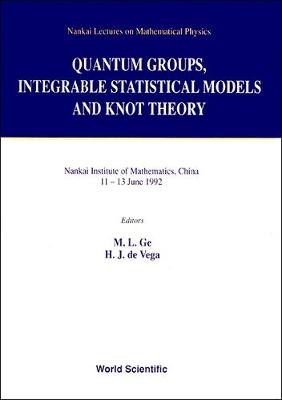•# Quantum Groups, Integrable Statistical Models And Knot Theory - The Fifth Nankai Workshop - Nankai Lectures On Mathematical Physics (Hardback)

(editor), (editor)
£88.00
Hardback 352 Pages / Published: 01/06/1993
• We can order this

Usually dispatched within 3 weeks

The lectures in this volume discuss topics in statistical mechanics, the geometric and algebraic approaches to q-deformation theories, two-dimensional gravity and related problems of mathematical physics, including Vassiliev invariants and the Jones polynomials, the R-matrix with Z-symmetry, reflection equations and quantum algebra, W-geometry, braid linear algebra, holomorphic q-difference systems and q-Poincare algebra.

Publisher: World Scientific Publishing Co Pte Ltd
ISBN: 9789810214746
Number of pages: 352